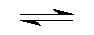## Hexa Aqua Potasium(+) Cation Complex Hydrolise  by Aris Kaksis Department of medical Biochemistry, Riga Stradin University RSU

At equilibrium, the following equilibrium equationis established withits conjugate base:

K+(H2O)6(aq)     +      H2O(l)K+(H2O)5(OH-)(aq)  + H3O+(aq)
acid extremely weak                                                       conjugatestrong base
The acid and its conjugate base have different colours. At low pH values the concentration of H3O+ is high and so
the equilibrium position lies to the left. The equilibrium solution has the colour A. At high pH values,the concentration
of H3O+is low - the equilibrium position thus lies to the right and the equilibrium solution has colour B.

blue (Acid)       pHhydrolize=12.75               red (Base)
KOH5HOHacdSM.skc   KOH5HOHacdSM.mol   KOH5HOHacdSM.tgf

We can apply equilibrium law to Brønsted equilibria- in general for a weak acid potasium complex:
Keq=( [H3O+]·[K+(H2O)5(OH-)]/([K+(H2O)6][H2O]))eq
Keq is known as the Brønsted equilibriaconstant. The pH for hydrolyse is calculated at this point when:
[K+(H2O)5(OH-)] =   [K+(H2O)6]
So from equation: Keq = [H3O+]/[H2O] =10-14.5/[H2O]= 10-14.5/55.3 = 210-16.24
Keq = [H3O+]/[H2O]; Keq[H2O]=[H3O+]=10-12.75;-log(Keq[H2O])=12.75 = pH = -log([H3O+])
The pH of the solution at its equilibrium point is called the pH andisthe pH at which half of the potasium is in its
acid form and the other half inthe form of its conjugate base.

At a low pH<14.5, a weak acid aqua complex of potasium ion is almostentirely in the [K+(H2O)6]form, the
concentration of which predominates. As the pH increases pH>14.5- theconcentration of [K+(H2O)6]decreases and
the equilibrium is pushed to the right to conjugatebase[K+(H2O)5(OH-)]form,the concentration of which predominates.

Aqua complex of potassium

We can apply equilibrium law to complexe stability constant equilibria- in general for a acid form potasium cation:

K+(aq)    + OH- (aq)K+(OH-)(aq)
blue (Acid)                             red (Base)
Kstab=[K+(OH-)]/([Na+][OH-])=10-0.5=([K+(OH-)][H3O+])/([K+][10-14])
Kstab is known as the Complexestability constant.The pH for hydrolyse is calculated at this point when:

[K+(OH-)] =   [K+]
So from equation: Kstab = [H3O+]/10-14 =10-0.5
Kstab10-14=[H3O+]=10-14.5; -log(Kstab10-14)= 14.5 = pH =-log([H3O+])
The pH of the solution at its equilibrium point is called the pH andisthe pH at which half of the potasium is in its
conjugate base and the other halfin the form of its acid form.

Complex maker of potasium cation
At a low pH<14.5 a weak acid aqua complex of potasium ion is almostentirely in the K+form free cation, the
concentration of which predominates. As the pH increases pH>14.5 -the concentration of [K+]decreases and the
equilibrium is pushed to the right to conjugatebase [K+(OH-)]form, the concentration of which predominates.

Literature

Handbook of  Chemical Equilibriain Analytical Chemistry.S.Kortly, L.Sucha. New York, Ellis HorwoodLTD. 1985. 414.p.